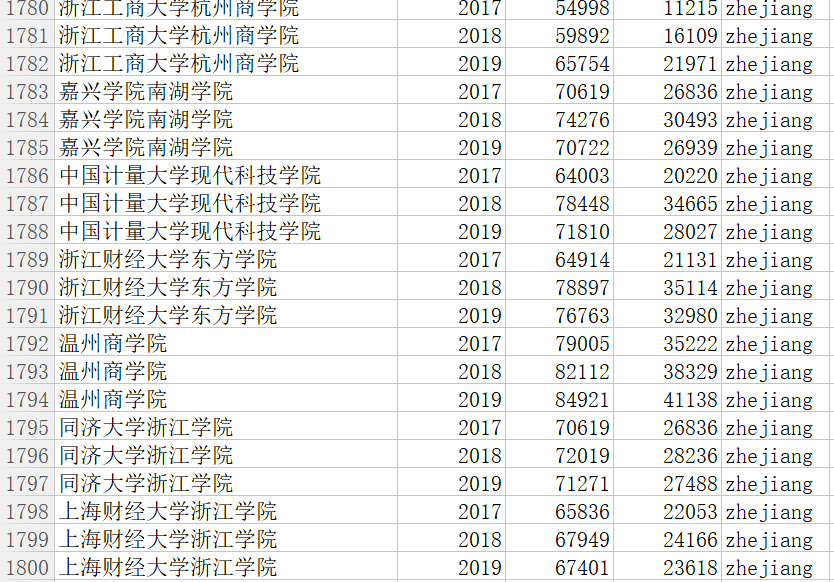# 高考填志愿：列一份娃娃能去的大学名单

Stata 连享会   主页 || 视频 || 推文

E-Mail: hnstedu@163.com

## 0. 使用说明

``````curriculum = "理科"     # 文科 或 理科
batchName  = "本科一批" # 本科一批 或 本科二批
province   = "福建"     # 考生所在省份
aim_score  = 43783      # 考生在本省的排名
``````

：目标高校筛选标准是根据近 5 年最低录取名次，只要考生排名至少 1 次在某高校最低录取名次之内，即认定为目标高校。

......## 1. 引言

https://gaokao.baidu.com/gaokao/m/school/home?word=清华大学 为例，选择福建省，在最低分排名目录下可以得到下图

## 2. 分析思路

`query`: 清华大学。用于学校识别。

`province`: 福建。用于省份识别。

`curriculum`: 理科。用于文理科识别。

`batchName`: 本科一批。用于批次识别。

Note：上述网址看似复杂，其实是因为中文字符被转码了，地址栏中显示的信息为：https://gaokao.baidu.com/gaokao/gkschool/scoreenroll?ajax=1&query=清华大学&province=福建&curriculum=理科&batchName=本科一批

## 3. R 代码实现

``````master_web = 'https://gaokao.baidu.com/gaokao/gkschool/scoreenroll?ajax=1&'
library(curl)
library(jsonlite)
##定义函数
score_fun <- function(query,province,curriculum,batchName){
firstp = curl_escape(query)
secondp = curl_escape(province)
thirdp = curl_escape(curriculum)
fourthp = curl_escape(batchName)
url = paste(master_web,'query=',firstp,'&province=',secondp,'&curriculum=',thirdp,'&batchName=',fourthp,sep="")
#print(url)
rating <- fromJSON(web)
flag = rating\$msg
if (flag=="没有符合条件的数据"){
print("Not OK")
}else{
data1 = rating\$data\$minScoreOrder
if (is.null(data1)==T) {
print("No")
}else{
pos = lapply(data1\$value,function(x) x\$value)
record = data.frame(school=query, year = data1\$key,score=unlist(pos))
}
}
}
``````

http://www.moe.gov.cn/mdcx/qggdxxmd/201912/t20191217_10000023.html#

``````##省份循环
setwd("D:\\school_choose") #设置工作路径
#考生相关信息
curriculum="理科"
batchName="本科一批"
province = "福建"

files = list.files(pattern=".csv")
for (i in 1:length(files)){ #省份循环
#for (i in 2:2){ #省份循环
filename1 = substr(files[i],1,nchar(files[i])-4)
filename = paste("D:\\school_choose\\result\\",filename1,"_run",".csv",sep="")
listresult = list()
query = dataf\$name
#province = gsub('(省|市)',"",dataf\$province)
for (j in 1:length(query)){ #省份内学校循环
print(query[j])
listresult[[j]] = score_fun(query[j],province,curriculum,batchName)
}
lresult = Filter(function(x) {is.null(nrow(x))==F}, listresult)
allresult = do.call(rbind,lresult)
write.csv(allresult,file=filename,row.names=F)
print(paste(filename1,"done"))
Sys.sleep(3)
}
``````

``````setwd("D:\\school_choose\\result")
m_result = list()
aim_score = 43783 #考生的排名位次
diff_score = 0
files = list.files(pattern=".csv")
for (i in 1:length(files)){
print(i)
file_name1 = substr(files[i],1,nchar(files[i])-8)
if (length(names(mydata))<=1) {
print("Not qualified")
}else{
#subdata = subset(mydata,year!="Not qualified")
mydata\$diff = suppressWarnings(as.numeric(as.vector(mydata\$score))) - aim_score
mydata\$p_name = file_name1
m_result[[i]] = subset(mydata,diff > diff_score)
}
}
mlresult = Filter(function(x) {is.null(nrow(x))==F}, m_result)
m_result1 = do.call(rbind,mlresult)
aim_file = paste("D:\\school_choose\\school\\","f_二批",".csv",sep="")
write.csv(m_result1,file=aim_file,row.names=F)

``````

## 6. 免责声明

### 附录

``````master_web = 'https://gaokao.baidu.com/gaokao/gkschool/scoreenroll?ajax=1&'
library(curl)
library(jsonlite)
##定义函数
score_fun <- function(query,province,curriculum,batchName){
firstp = curl_escape(query)
secondp = curl_escape(province)
thirdp = curl_escape(curriculum)
fourthp = curl_escape(batchName)
url = paste(master_web,'query=',firstp,'&province=',secondp,'&curriculum=',thirdp,'&batchName=',fourthp,sep="")
rating <- fromJSON(web)
flag = rating\$msg
if (flag=="没有符合条件的数据"){
print("Not OK")
}else{
data1 = rating\$data\$minScoreOrder
if (is.null(data1)==T) {
print("No")
}else{
pos = lapply(data1\$value,function(x) x\$value)
record = data.frame(school=query, year = data1\$key,score=unlist(pos))
}
}
}
``````

``````##省份循环
setwd("D:\\school_choose") #设置工作路径
#考生相关信息
curriculum="理科" #根据考生实际情况修改：文科或理科
batchName="本科一批" #根据考生实际情况修改：本科一批或本科二批
province = "福建" #根据考生实际情况修改：考生所在省份

files = list.files(pattern=".csv")
for (i in 1:length(files)){ #省份循环
filename1 = substr(files[i],1,nchar(files[i])-4)
filename = paste("D:\\school_choose\\result\\",filename1,"_run",".csv",sep="")
listresult = list()
query = dataf\$name
for (j in 1:length(query)){ #省份内学校循环
print(query[j])
listresult[[j]] = score_fun(query[j],province,curriculum,batchName)
}
lresult = Filter(function(x) {is.null(nrow(x))==F}, listresult)
allresult = do.call(rbind,lresult)
write.csv(allresult,file=filename,row.names=F)
print(paste(filename1,"done"))
Sys.sleep(3)
}
``````

``````setwd("D:\\school_choose\\result")
aim_score = 43783  #根据考生实际情况修改：考生在本省的排名
m_result = list()
files = list.files(pattern=".csv")
for (i in 1:length(files)){
print(i)
file_name1 = substr(files[i],1,nchar(files[i])-8)
if (length(names(mydata))<=1) {
print("Not qualified")
}else{
#subdata = subset(mydata,year!="Not qualified")
mydata\$diff = suppressWarnings(as.numeric(as.vector(mydata\$score))) - aim_score
mydata\$p_name = file_name1
m_result[[i]] = subset(mydata,diff > 0)
}
}
mlresult = Filter(function(x) {is.null(nrow(x))==F}, m_result)
m_result1 = do.call(rbind,mlresult)
aim_file = paste("D:\\school_choose\\school\\","f_二批",".csv",sep="")
write.csv(m_result1,file=aim_file,row.names=F)
``````

## 相关课程

http://lianxh.duanshu.com

### 课程一览

Stata数据清洗 游万海 直播, 2 小时，已上线

Note: 部分课程的资料，PPT 等可以前往 连享会-直播课 主页查看，下载。

#### 关于我们

• Stata连享会 由中山大学连玉君老师团队创办，定期分享实证分析经验。直播间 有很多视频课程，可以随时观看。
• 连享会-主页知乎专栏，300+ 推文，实证分析不再抓狂。
• 公众号推文分类： 计量专题 | 分类推文 | 资源工具。推文分成 内生性 | 空间计量 | 时序面板 | 结果输出 | 交乘调节 五类，主流方法介绍一目了然：DID, RDD, IV, GMM, FE, Probit 等。
• 公众号关键词搜索/回复 功能已经上线。大家可以在公众号左下角点击键盘图标，输入简要关键词，以便快速呈现历史推文，获取工具软件和数据下载。常见关键词：`课程, 直播, 视频, 客服, 模型设定, 研究设计, stata, plus, 绘图, 编程, 面板, 论文重现, 可视化, RDD, DID, PSM, 合成控制法`

✏ 连享会学习群-常见问题解答汇总：
https://gitee.com/arlionn/WD

#### 热门资讯

12/05 计量专题 9696
10/10 专题课程 8530
01/20 内生性-因果推断 129
01/20 Stata教程 121
01/20 Stata命令 55